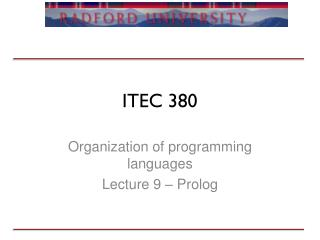DownloadDownload PresentationITEC 380

# ITEC 380

Télécharger la présentation## ITEC 380

- - - - - - - - - - - - - - - - - - - - - - - - - - - E N D - - - - - - - - - - - - - - - - - - - - - - - - - - -
##### Presentation Transcript

1. ITEC 380 Organization of programming languages Lecture 9 – Prolog

2. Review • Prolog • What are its 2 major components? • Where are facts stored? What is their relationship with the interpreter? • What is the difference between upper and lower? • User I/O • Email me a 3rd generation OO language by the end of the week or I’ll pick one!

3. Objectives • Homework 2 • Prolog • Recursion • Lists

4. Notes • When you see …/2 in prolog that means a rule that takes 2 parameters • Is variable, and lets you know what the rule expects if there is an issue • Can have multiple rules, facts with same name • Friend(X) :- Person1(X). • Friend(X) :- Person2(X).

5. Exercise • Time for a bit of magic • There is a problem with this example, can you spot it? house_elf(dobby).    witch(hermione).    witch(’McGonagall’).    witch(rita_skeeter).    magic(X):-  house_elf(X).    magic(X):-  wizard(X).    magic(X):-  witch(X).

6. Exercise • What is the difference between • read(r), X is r * r, write(X). • read(R), X is R * R, write(X). • Simple calculations • The area of a circle is 2*pi*r • Pythagoras (given a and b [sides], what is c [hypotenuse]

7. Recursion • What is recursion? • What are the benefits? • What are the downsides? • Prolog syntax • rule(parameters) :- rule(parameters), baseCase(parameters). • Remember • Always use the base case

8. Example • Descendants • Anne has a daughter named Bridget • Bridget has a daughter named Caroline • Caroline has a daughter named Donna • Donna has a daughter named Emily • How would you represent this information? • How would you specify the ability to query if a person is a descendant of another? • What are some uses for this type of query?

9. Question • What is the difference between child(anne,bridget). child(bridget,caroline). child(caroline,donna). child(donna,emily). descend(X,Y) :- descend(Z,Y), child(X,Z). descend(X,Y) :- child(X,Y). and descend(X,Y) :- child(X,Y). descend(X,Y) :- descend(Z,Y), child(X,Z).

10. Caution • Run away stacks • Always recurses in the order of rules • Must make sure you stop! • What about bad input? • Very easy to cause an infinite loop through bad queries • One of the most common problems, limiting factors of prolog

11. Lists • In prolog you use [ ] to denote a list • List items are separated by ,’s • Lists can contain lists • Can query certain parts of the list like Lisp • For example • [Head|Tail] = [apple, pear, peach]. • What do you think Head contains? What about Tail? • Exercise • Write a function to give you the third item in a list

12. Contents • Empty list is [ ], can be checked with Var = [ ] • What happens if you combine not(Var = [ ]) in a rule? • Can do more than [ X | Y ], for example [ X,Y,Z | W ] • Can append data with append(list1,list2, Var) • Allows you to build up a list • Can figure out length with Length(list,X). • Member(X,[list]) returns with value or true/false depending on variable or constant

13. Examples • PrintList(L). %Print the contents of the list • reversePrint(L). %Print the contents of the list in reverse • revList(L). %Reverse a list • Palindrome checker • Is a word spelled the same forwards / backwards

14. Exercise • How would you create a stack that allows users to add values to it? • How would you use this stack with a command that will add the 2 top numbers on the stack (removing them and adding the result to the top of the stack)? • How do we tie this in so it allows commands to be entered multiple times?

15. Methods of reversing • Accumulator versus appending • How do we tell which one is better? naiverev([],[]). naiverev([H|T],R):-  naiverev(T,RevT),  append(RevT,[H],R). Versus accRev([H|T],A,R):-  accRev(T,[H|A],R). accRev([],A,A). rev(L,R):-  accRev(L,[],R).

16. Next week • More in-depth with prolog • Graphics